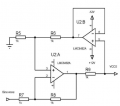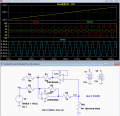# How does a two amplifier VCCS (voltage controlled current source) work?

#### roxi60

Joined Sep 2, 2018
73Hello.
Can someone please explain, or indicate where I can find the detailed working principle explanation, on how a circuit like this can provide a constant current, when generally a voltage (not necessarily sine wave as indicated, but also a constant voltage) is applied before R7?
For instance, among the others, why the feedback voltage on non inverting pin is the middle of a partition between R5 and R6?

Thank you so much.

#### crutschow

Joined Mar 14, 2008
32,026
The circuit is basically an improved Howland current source with an added op amp buffer (U1a) in the positive part of the feedback loop to eliminate the error in the output current due to the current through R1 and R4 (my circuit below).
The circuit uses positive feedback from the output load so that the output of U1b increases with the load resistance, and thus maintains a constant current.
The feedback is taken from the divider of R1 and R4 so that the feedback is just the right amount to correct for the increase in load voltage with resistance.
(Edit: Another way to look at it, is that the circuit maintains a constant voltage across R3 independent of the load resistance.)
It basically looks rather like a differential circuit which works as long as R2=R4, and R1=R5.
Changing the overall ratio of these two sets of resistors will change the gain of the circuit.

The LTspice simulation below, shows how the output current is constant (blue trace) as the output load resistance increases (yellow trace) for a constant input voltage.Last edited:
•Mr.1980

#### roxi60

Joined Sep 2, 2018
73
The circuit is basically an improved Howland current source with an added op amp buffer (U1a) in the positive part of the feedback loop to eliminate the error in the output current due to the current through R1 and R4 (my circuit below).
The circuit uses positive feedback from the output load so that the output of U1b increases with the load resistance, and thus maintains a constant current.
The feedback is taken from the divider of R1 and R4 so that the feedback is just the right amount to correct for the increase in load voltage with resistance.
It basically looks rather like a differential circuit which works as long as R2=R4, and R1=R5.
Changing the overall ratio of these two sets of resistors will change the gain of the circuit.

The LTspice simulation below, shows how the output current is constant (blue trace) as the output load resistance increases (yellow trace) for a constant input voltage.

View attachment 195018
Thank you very much,Crutshow, for your kind explanation and for the example!

#### crutschow

Joined Mar 14, 2008
32,026
One thing I forgot to mention is that you can connect the input signal to R4 while grounding R2, which will make the input to output phase non-inverting rather than inverting.
Basically those two inputs are differential.

If you are interested in analog design, I suggest you download the free LTspice simulator from Analog Devices, which several on these forums use.
It has a somewhat steep learning curve, but there are good tutorials and included circuit examples so ease the process, as well as help here with any questions.

Last edited:
•Mr.1980